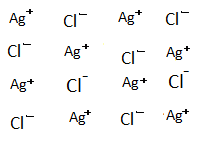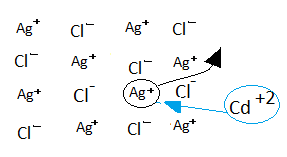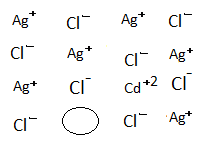# What happens when AgCl is doped with $CdC{l_2}$? What is the name of this defect?Verified
198.9k+ views
Hint: The defects occur in crystals due to the presence of cationic or anionic vacancies in the lattice points or dissociation of ions in the lattice. This imperfection is known as a defect.

Complete step by step answer:
-The constituent ions in AgCl and $CdC{l_2}$ are $A{g^ + }$,$C{l^ - }$,$C{d^{ + 2}}$. Here doping means basically the addition of $CdC{l_2}$ to AgCl.-When AgCl is doped with $CdC{l_2}$.-One of $A{g^ + }$ ion is replaced by $C{d^{ + 2}}$ ion after which, in order to maintain the crystal electrically neutral another $A{g^ + }$ ion moves out leaving a hole.
-Like this, the number of holes is created thus; increases the conductance due to the movement of $A{g^ + }$ ion.
-The imperfection in the crystals implies the imperfect arrangement of constituent ions is due to the presence of cationic or anionic vacancies in the lattice points or dissociation of ions in the lattice. This imperfection is known as a defect.
-The defect seen in this case is an impurity defect and vacancy defect.
-Impurity defect can be defined as the defect that is created due to the replacement of the actual ion by some foreign ion, in consequence of this many intrinsic holes are created. This exactly meets our explanation given above. Therefore, impurity defect can be seen in this case.
-As many holes are created due movement of $A{g^ + }$. Thus this case is exactly apt to vacancy defects also.

Note: points to remember:
- Increases the conductance due to movement of $A{g^ + }$ ion: As increase in the number of holes created by movement of $A{g^ + }$ ion, the conductance also increases in the same trend.
- Impurity defect as well as vacancy defect are seen in this case.